Mathematics

# Operations and Algebraic Thinking Grade 4

1.3k views

 1 Introduction 2 What is Algebraic Thinking? 3 Algebraic thinking for Grade 4: Patterns and Operations 4 Examples 5 Operations and Algebraic Thinking Grade 4 Worksheet 6 Summary 7 FAQs

22 December 2020

## Introduction

When children start to explore and learn about patterns, it makes them build important foundations for later work on number operations. Algebraic patterns help children build a strong math foundation by creating, extending, naming, and talking.

Patterns are at the heart of math. The ability to recognize and create patterns helps children make predictions based on their observations; this is an important math skill. Understanding patterns help prepare children for learning complex number concepts and mathematical operations.

In this article, the need for algebraic thinking in grade 4 students and what kind of activities aid in developing this most required learning formulation could be seen.

## Operations and Algebraic Thinking Grade 4 - PDF

If you ever want to read it again as many times as you want, here is a downloadable PDF to explore more.

## What is Algebraic Thinking?

To ensure students succeed at the formal study of algebra, algebraic thinking would be instrumental in improving their abilities.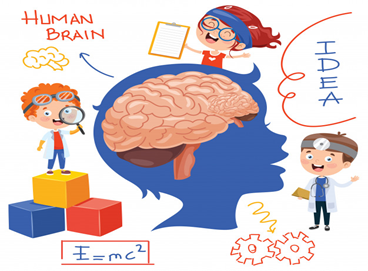Algebraic thinking includes

• Perceiving and investigating patterns,
• Studying and exhibiting relationships,
• Making conceptions
• Evaluating how things change.

It is essential to understand algebraic symbols.

At the most earlier grades, little youngsters work with designs. Kids have a characteristic love of mathematics during this early age. Their interest is helps as they attempt to portray and expand examples of shapes, colors, sounds, and in the end, letters and numbers.

Furthermore, kids start to generalize examples that appear to be the equivalent or unique at a young age. This sort of classifying and summing up is a significant formative advance on the excursion towards algebraic thinking.

In the above video Arjun, a 3rd-grade Cuemath student, explains to us how he learned to count and recognize patterns at Cuemath.

## Algebraic thinking for Grade 4: Patterns and Operations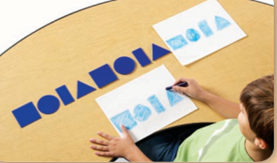Math Patterns are sequences that repeat based on a rule, and a rule is a set way to calculate or solve a problem. All through the initial assessments, patterns are an object of study as well as a mechanism too.

As students build up their comprehension of numbers, they can utilize patterns in varieties of spots or have a problem to perceive what 5 is or whether 6 is more significant than 8.

Looking at what is the same and what is different among functions lies at the heart of understanding algebraic skills and processes.

In grade 4, students investigate and learn addition, subtraction, multiplication, and division. They can search for designs that assist them with learning systems and realities.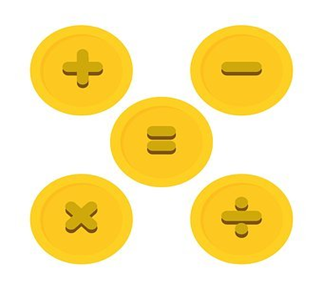They understand remainders and quotients in division. They utilize mental calculation and assessment techniques.

Students likewise find out about factors and multiples of numbers. They understand prime and composite.

Also, they learn and apply ideas that will help assemble an establishment critical for later work in Algebra. They identify patterns in generating outputs with rules. Such understanding will help them be successful with later algebraic functions and equations.

## Examples

### 1. Comparing and ordering objects by weight

It allows students to apply their understanding of greater than and less than in a new context.

Finding the amount of an object that equals the weight of another by removing the same amount from both sides of a balance prepares them for later understanding algebraic expressions.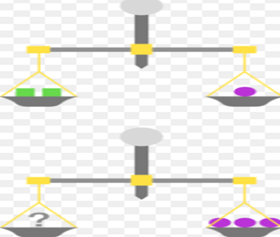Example

Find out the number of squares to be placed to balance the scale.

Solution

Here we use a balance to compare the quantities of two objects. As shown in the figure, one circle is equal to two squares, so what will be the weight of three circles.

1 circle = 2 squares

\begin{align}\text{Therefore 3 circles }&= 3 \times 2 \text{squares}\\&= 6 \text{squares}\end{align}

6 squares are to be placed on the left side of the balance.

### 2. Finding factor pairs

Discovering factor sets permits students to develop their grasp of division as they find out about factors and divisibility rules. To find all the factor sets of a number, students should be asked to proceed in a figured out way by finding the factor pair of 1, then by 2, 3, 4, and so forth.

 Example

Write each multiplication fact and use Fraction rods to build each number and factor pairs shown.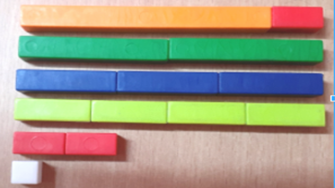1. 12

Solution

Factor pairs are

\begin{align}1 × 12 = 12\\2 × 6 = 12\\3 × 4 = 12\\4 × 3 = 12\\6 × 2 = 12\\12 × 1 = 12\end{align}

### 3. Determine if a number is prime or composite

Students finding out about factors find that a few numbers have less factors than different numbers. A prime number has precisely two factors: the number 1 and the number itself. A composite number has multiple factors. Since the number 1 has just itself as a factor, it is neither prime nor composite.

 Example

Use Color Tiles to model each number. Write the number. Is the number prime or composite?

1. 9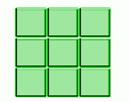Here the 3 × 3 grid is formed, so 9 is composite because 1, 3 and 9 are factors.

1. 3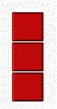Here the 1 × 3 grid is formed, so 3 is prime because 1 and the number itself are the only factors.

### 4. Identifying Multiples and Division facts

A grasp of multiplication is helpful to students when they work with multiplication, division, and equivalent fractions. Additionally, they need to comprehend multiples to discover the least common multiples and most significant highest common factors. A considerable amount of time should be committed to building up youngsters' comprehension of multiplication and how it functions. Time invested in doing this is very much spent. Hence, kids become more sure with their understanding of multiplication before they proceed with their excursion into the multiplication table and tackling multiplication problems.

 Example 1

Answer the questions that describe the model.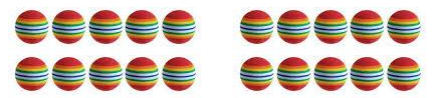1. How many groups are there?

2 groups

2. How many balls are there in each group ?

10 balls

3. Write a multiplication sentence to describe the model.

Multiplication fact = 2 × 10 = 20

4. How many balls are there altogether?

 This can be written as $$2 \times 10$$ $$= 20$$ Which can be written as $$2 × 10$$ $$= 20$$ Number of groups Number of balls No of balls per group
 Example 2

Use Color Tiles. Build the array. Answer the questions.

Is 18 a multiple of 6 ? Why or why not?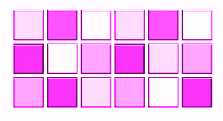Solution

Yes, 18 is a multiple of 6 because there can be 6 rows of 3 or 3 rows of 6 as shown in the grid.

Multiplication fact = 6 × 3 = 3 × 6 = 18.

Division Facts

Solving a division problem is introduced as early as grade 2 by introducing division by distribution, division on the number line and writing multiplication and division statements.

and then they are introduced with long division and remainders. These concepts form the basis for generating the right division facts by students in grade 4. A few examples are given below for division by grouping and the division on the number line.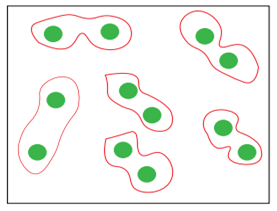Example 3

1. Fill the boxes

Solution

12 items are divided into 6 groups.

Each group has 2 items

Division fact: 12 ÷ 6 = 2

2. Divide using the number line.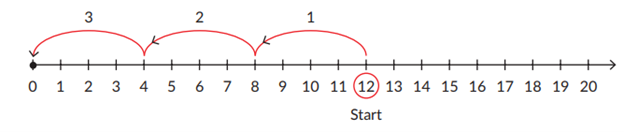Divide 12 into groups of 4.

Division fact: 12 ÷ 4 = 3

### 5. Extend patterns

Students should be able to express descriptions of patterns in words and in numbers. Students who understand a pattern will be able to extend the pattern.

 Example

Use Attribute Blocks to model each pattern. Describe the repeating part. How many times does it repeat?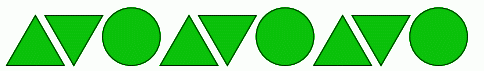Solution

Repeating part triangle, upside down triangle, circle

Number of times repeated 3

## Operations and Algebraic Thinking Grade 4 Worksheet

G4.W1.M1.S1 Date :

1. Which Picture is Correct?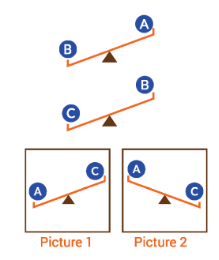1. Circle the factors of the given number.

Number

Factors of the number

14

1 , 28 , 15 , 7 , 21 , 2 , 14

8

1 , 16 , 7 , 2 , 12 , 4 , 6 , 24

12

12 , 6 , 8 , 24 , 3 , 4 , 2 , 1

20

1 , 5 , 20 , 15 , 10 , 2 , 3 , 5

24

1 , 3 , 6 , 12 , 8 , 2 , 4

18

2 , 6 , 12 , 9 , 18 , 1 , 15 , 3

1. Tick (✓) all the options that are correct.

A prime number has only two factors, 1 and the number itself.

A prime number is a multiple of only two numbers, 1 and the number itself.

Some prime numbers have more than two factors.

1. Find the number of dots in the dot array.

a.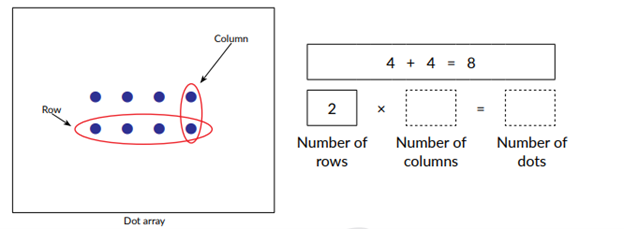b.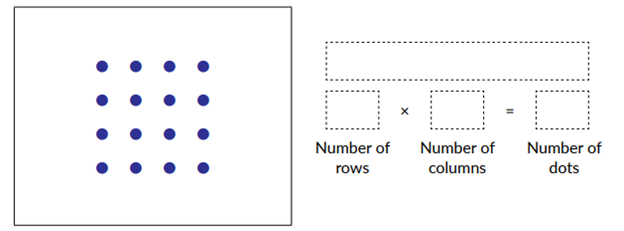1. Complete the picture for the problem and find the answer.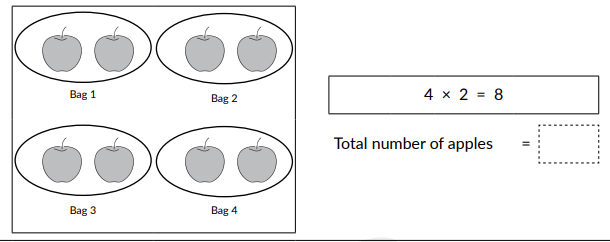2. Draw a picture for the problem and find the answer.

Riya bought three chocolate boxes. Each box has eight chocolate pieces. How many chocolate pieces did she buy?

1. Group the circles for the given division facts and fill the boxes.

a.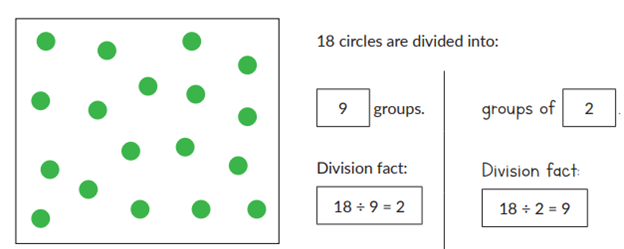b.  Divide 12 circles into 4 groups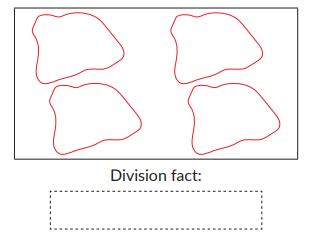1. Which object is missing? Tick (✓) the correct option

a.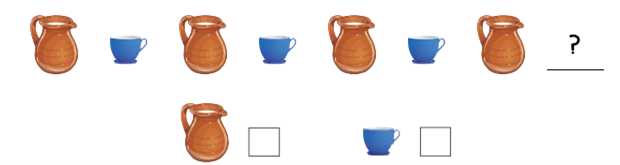b.## Summary

The improvement of algebraic reasoning is a pattern, not a phenomenon. It is something that can be essential for a constructive, spurring, enhancing school mathematics experience.

Students must stay motivated and not give up while solving complex problems. The curriculum must be structured to help young learners.

Cuemath learning tools enable children to calculate numbers and recognize patterns mentally. This method of visual learning is very useful for children. They can actually visualize numbers, patterns, and shapes. And, in the process, increase their speed and accuracy and become proficient in solving algebraic problems.

Make sure to practice regularly, almost every day for at least 30 to 45 minutes,  get better at math with Cuemath, sign up for a free trial

Written by Jesy Margaret, Cuemath teacher

Cuemath, a student-friendly mathematics and coding platform, conducts regular Online Live Classes for academics and skill-development, and their Mental Math App, on both iOS and Android, is a one-stop solution for kids to develop multiple skills. Understand the Cuemath Fee structure and sign up for a free trial.

## What is algebraic thinking?

Algebraic thinking includes:

• Perceiving and investigating patterns
• Studying and exhibiting relationships
• Making conceptions
• Evaluating how things change

The benefit in using algebraic symbols is an integral part of becoming proficient in applying algebra to solve problems.

## How to learn algebraic thinking?

Algebraic thinking can be learnt with the following techniques:

• Students realize the tangible and symbolic representations relating to conditions and solve multi-step word problems utilizing the four tasks: addition, subtraction, multiplication, and division
• They decipher what remainder means inside this present reality setting of division problems. They utilize mental calculation and assessment techniques
• Students likewise find out about factors and multiples of numbers. They come to realize the terms prime and composite
• Also, they learn and apply ideas that will help assemble a foundation important for later work in Algebra. They identify patterns in generating outputs with rules. Such understanding will help them be successful with later algebraic functions and equations

Award-winning math curriculum, FREE for a year